## Quiz 18 : Pricing and Profitability AnalysisLooking for Accounting Homework Help?# Quiz 18 : Pricing and Profitability Analysis

1.The Mark Up Percentage shall include all the cost that are not the part of cost of goods sold and thereafter desired profit is added, it is calculated using formula: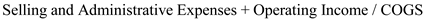Where, COGS means COST OF GOODS SOLD. Hence applying the above formula we get:2.The price of new product shall be calculated in the following manner:3.Mark up on Direct Material shall be calculated in following manner:Hence, applying the above formula we get;Note: All the values are in \$

Price elasticity of demand: • It means percentage of change in demand of the product due to change in particular price for its price. • It is calculated as the change in quantity percentage of the product divided by the change in price of that product. • If demand is relatively elastic, a small price change will result a higher percentage of quantity demand of that product change. The opposite is true for inelastic demand. " Shirts and trousers available at malls " example for relative elasticity demand and " grocery items" available at super markets and "vegetables" are best examples for the inelastic demand.

1. The total cost per case for each of the 3 customers shall be calculated in following manner: Super Markets: The cost shall be calculated in following manner: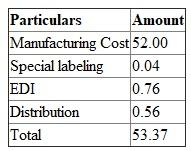Explanations: a. Price per case is \$ 52 b. Labeling cost is.04 c. EDI cost is calculated in following manner:The EDI cost is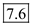d. The distribution costs are calculated in following manner:Hence, the distribution cost is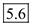Small Grocers: The calculation shall be made in following manner: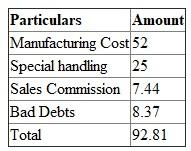Explanations: a. Price Per case is \$ 52 b. Special handling cost is \$ 25 c. Sales Commission is calculated in following manner:d. The Bad debts are calculated in following manner:Convenience Stores: The Calculation shall be made in following manner: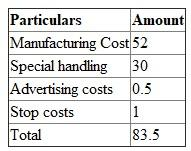Explanations: a. Price Per case is \$ 52 b. Special handling cost is \$ 30 c. Sales Commission is calculated in following manner:d. The Stop Costs are calculated in following manner:2. The profit per case shall be calculated as a difference between sales price and cost price hence it shall be calculated in following manner: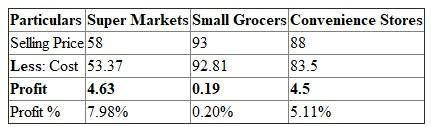Profit % for supermarkets:Profit % for small grocers:Profit % for convenience stores:3. The Average selling price is \$ 79.67 and if average price is charged from each customer then in that case the profits in super markets will increase significantly while the profits in small grocers and convenience stores will fall down and henceforth the profit percentage will fall for small grocery and convenience stores.

There is no answer for this question

There is no answer for this question

There is no answer for this question

There is no answer for this question